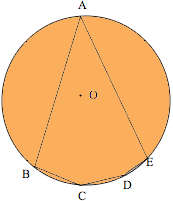SEARCH HOMEMath Central Quandaries & QueriesO is the center of the circle.  Sides AB and AE are equal.  Angle B = 95, angle C = 130, angle D = 138.  Find angles A and E. FaisalFaisal's diagram

Faisal,

The theorem you should try to use here:

BCDE is a quadrilateral inscribed in a circle so the sum of the angles at B and D add up to 180 degrees.

To set the theorem up, set the angle at A equal to x degrees. Then in the isosceles triangle ABE you can figure out the measure of the equal angles at B and E in terms of x. Subtract that number from the total angle of the pentagon at B (namely 95 degrees) and you get the portion of the angle B that lies in the quadrilateral BCDE. Add the resulting number to angle D = 135; the total must be 180 degrees, so you have an equation that you can solve for x.

ChrisMath Central is supported by the University of Regina and The Pacific Institute for the Mathematical Sciences.